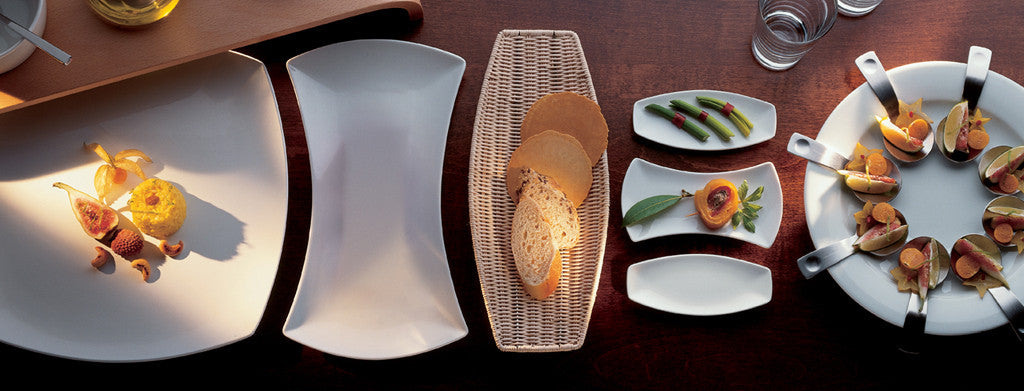Shopping Cart

### Refine

View all

#### Price# Crockery£4.69 each (ex VAT)£2.74 each (ex VAT)£3.25 each (ex VAT)£8.17 each (ex VAT)£6.30 each (ex VAT)£3.54 each (ex VAT)£4.83 each (ex VAT)£5.93 each (ex VAT)£17.56 each (ex VAT)£21.51 each (ex VAT)£22.69 each (ex VAT)£3.81 each (ex VAT)£1.10 each (ex VAT)£1.22 each (ex VAT)£3.83 each (ex VAT)£3.23 each (ex VAT)£16.17 each (ex VAT)£3.26 each (ex VAT)£5.12 each (ex VAT)£3.47 each (ex VAT)£5.98 each (ex VAT)£10.03 each (ex VAT)£14.03 each (ex VAT)£3.39 each (ex VAT)£8.73 each (ex VAT)£6.04 each (ex VAT)£18.32 each (ex VAT)£18.10 each (ex VAT)£1.95 each (ex VAT)£4.27 each (ex VAT)£2.99 each (ex VAT)£2.07 each (ex VAT)£2.96 each (ex VAT)£5.54 each (ex VAT)£3.14 each (ex VAT)£2.42 each (ex VAT)£3.26 each (ex VAT)£9.93 each (ex VAT)£4.66 each (ex VAT)£3.50 each (ex VAT)£3.88 each (ex VAT)£7.62 each (ex VAT)£3.45 each (ex VAT)£28.68 each (ex VAT)£4.32 each (ex VAT)£5.45 each (ex VAT)£5.08 each (ex VAT)£5.36 each (ex VAT)